#DAY 10
3
Modern Web

## Day10-用C#算數學!(基本運算、平方、平方根、log、sin)

1.輸入x,y，計算(x+y)/2
2.輸入x,y，計算x²-y²
3.輸入x，計算y=√?
4.輸入x，計算log(x)、log(a,10)、log10(a)
5.輸入x，計算y=sin(x)

### 1.輸入x,y，計算(x+y)/2

`````` int x, y;//宣告兩個變數
Console.Write("請輸入 x:");//在螢幕上show出請輸入 x:
//但是x是int，無法儲存string的值，所以這裡我們將string轉型為int
Console.Write("請輸入 y:");
Console.WriteLine("x = {0}, y={1},答案={2}", x,y,(x+y)/2);
//這裡也可以宣告一個新變數接x+y的值，例如z=(x+y)/2,Console.WriteLine("x = {0}, y={1},答案={2}", x,y,z);
``````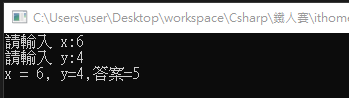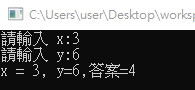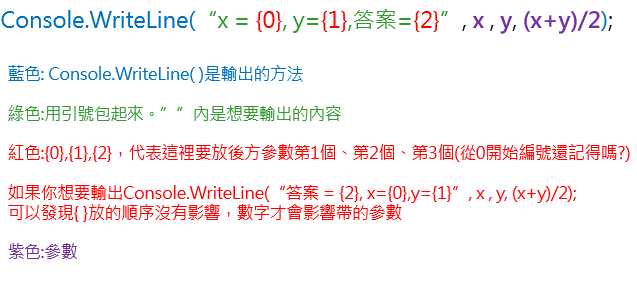``````Console.WriteLine("x ="+x+", y="+y+",答案="+(x+y)/2);
``````

================================================

2.另外目前如果想要字串中插入變數，在C#6.0之後，提供了string interpolation(字串插補)方法，可以在字串宣告前方加入 \$ 符號，搭配{ }，就可以直接用插值的方式傳入變數。

``````Console.WriteLine("x = {0}, y={1},答案={2}", x,y,(x+y)/2);
//等同於
Console.WriteLine(\$"x = {x}, y={y},答案={(x+y)/2}");
``````

=================================================

### 2.輸入x,y，計算x²-y²

``````double a, b;
Console.WriteLine("以下計算a平方-b平方");//輸出此段文字並換行
Console.Write("請輸入 a = ");//輸出此段格式
Console.Write("請輸入 b = ");
Console.WriteLine("a平方-b平方={0}", Math.Pow(a, 2)-Math.Pow(b,2));
``````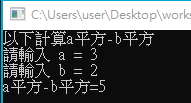### 3.輸入x，計算y=√?

1. 使用上方求次方的方式，根號=1/2次方=0.5次方
``````double a;
Console.WriteLine("求a開根號");
Console.Write("請輸入 a = ");
Console.WriteLine("a開根號={0}", Math.Pow(a,0.5));
``````
1. 使用Math.Sqrt()方法
``````double a;
Console.WriteLine("求a開根號");
Console.Write("請輸入 a = ");
Console.WriteLine("a開根號={0}", Math.Sqrt(a));
``````

Math.Sqrt(double 指定數字)

### 4.輸入x，計算log(x)、log(a,10)、log10(a)

``````Console.WriteLine("求log(a) 及 log(a,10) 及log10(a)");
Console.Write("請輸入 a = ");
Console.WriteLine("log(a)={0} 及 log(a,10)={1} 及 log10(a)={2}", Math.Log(number), Math.Log(number, 10), Math.Log10(number));
``````

log這裡用的是Math.Log的方法，跟log相關有四種使用方法
Math.Log(double x)以e = 2.718為底
Math.Log(double 數字,double 底數)以後方數字為底
Math.Log10(double x)，以10為底
Math.Log2(double x)，以2為底

### 5.輸入x，計算y=sin(x)

``````double a;
Console.WriteLine("求sin(a)");
Console.Write("請輸入 a = ");
Console.WriteLine("sin(a)={0}", Math.Sin(a));
``````

Math.Sin(double x)
1.x必須是弧度，但我們熟悉的計算是角度，角度跟弧度換算公式為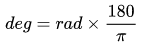pi可以使用Math.PI，C#的 π 精確到小數點後16位
2.a值範圍從大約-9223372036854775295 到大約9223372036854775295，超出此範圍會原封不動傳回，不會報錯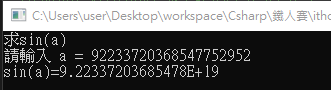cos傳送門
tan傳送門

## 參考資料

MSDN-Math Class

### 1 則留言

1

...((太多了，中間略過~XDDD

``````string a = "Hello";
string b = "World";
string formatAB = String.Format("{0} ~ {1}", a, b);
Console.WriteLine(formatAB); // 輸出: Hello ~ World
// 結果等同於
Console.WriteLine("{0} ~ {1}", a, b); // 輸出: Hello ~ World
``````

``````string a = "Hello";
string b = "World";
string before = String.Format("{0} ~ {1}", a, b);
string now = \$"{a} ~ {b}";

Console.WriteLine("{0} ~ {1}", a, b); // 輸出: Hello ~ World
Console.WriteLine(before); // 輸出: Hello ~ World
Console.WriteLine(now); // 輸出: Hello ~ World
``````

\$超方便XDD!!居然忘記介紹它了XD立馬補充!